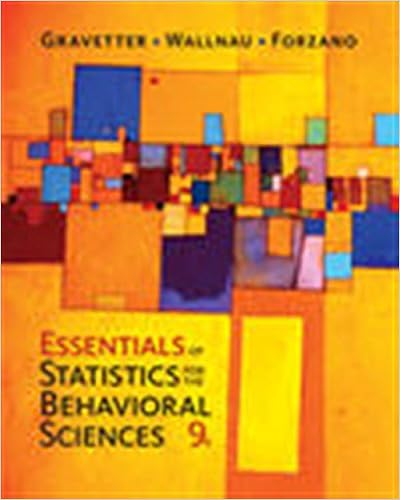# I a variable whose possible outcomes are coded as a 1

• Test Prep
• 72
• 90% (29) 26 out of 29 people found this document helpful

This preview shows page 22 - 24 out of 72 pages.

##### We have textbook solutions for you!
The document you are viewing contains questions related to this textbook.The document you are viewing contains questions related to this textbook.
Chapter 15 / Exercise 1
Essentials of Statistics for the Behavioral Sciences
Gravetter/WallnauExpert Verified
71.i. A variable whose possible outcomes are coded as a "1" or a "0" is called a dummy variable.ii. A dummy variable is added to the regression equation to control for error.iii. If the null hypothesis β4= 0 is not rejected, then the independent variable X4has no effect in predicting the dependent variable. A. (i), (ii) and (iii) are all correct statementsB. (i) and (ii) are correct statements, but not (iii).C. (i) and (iii) are correct statements but not (ii).D. (ii) and (iii) are correct statements but not (i).E. All statements are false
72.i. A variable whose possible outcomes are coded as a "1" or a "0" is called a dummy variable.ii. If the null hypothesis β4= 0 is not rejected, then the independent variable X4has no effect in predicting the dependent variable.iii. A dummy variable is added to the regression equation to control for error.
73.What can we conclude if the net regression coefficients in the population are not significantly different from zero?
74.Hypotheses concerning individual regression coefficients are tested using which statistic?
H
75.The best example of a null hypothesis for testing an individual regression coefficient is: A. H0: β1= β2= β3= B. H0: μ1= μ2= μ3= C. H0: β1= 0D. If F is greater than 20.00 then reject
β4
μ4
##### We have textbook solutions for you!
The document you are viewing contains questions related to this textbook.The document you are viewing contains questions related to this textbook.
Chapter 15 / Exercise 1
Essentials of Statistics for the Behavioral Sciences
Gravetter/WallnauExpert Verified
76.Angela Chou has been asked to investigate the determinants of poverty in Ontario communities. She collected data on 60 communities from Statistics Canada. She selected the percentage of poor persons living under the poverty line [Poor (%)], measured by Low Income Cut-Off, designed by Statistics Canada as a measure of poverty for a community, as the dependent variable. The independent variables selected are percent of single families in each community, the unemployment rate in each community, percent of population in the community holding a bachelor's degree as their highest level of education attained, and percent of population holding a High School Diploma as their highest level of education attained. Using the outputs below for this data set, determine whether Angela should use the model with the high school data included, or the data without the high school data, and why.
•••